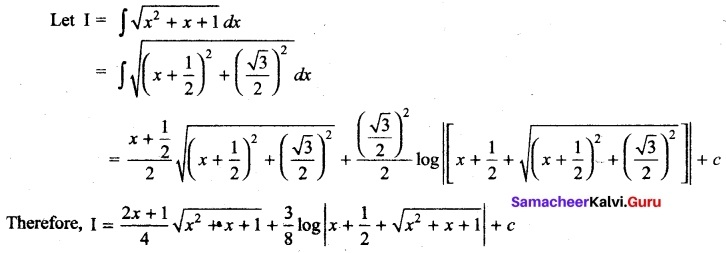# Tamil Nadu 11th Maths Previous Year Question Paper March 2019 English Medium

Students can Download Tamil Nadu 11th Maths Previous Year Question Paper March 2019 English Medium Pdf, Tamil Nadu 11th Maths Model Question Papers helps you to revise the complete Tamilnadu State Board New Syllabus and score more marks in your examinations.

## TN State Board 11th Maths Previous Year Question Paper March 2019 English Medium

General Instructions:

1. The question paper comprises of four parts.
2. You are to attempt all the parts. An internal choice of questions is provided wherever applicable.
3. All questions of Part I, II, III and IV are to be attempted separately.
4. Question numbers 1 to 20 in Part I are Multiple Choice Questions of one mark each.
These are to be answered by choosing the most suitable answer from the given four alternatives and writing the option code and the corresponding answer
5. Question numbers 21 to 30 in Part II are two-mark questions. These are to be answered in about one or two sentences.
6. Question numbers 31 to 40 in Part III are three-mark questions. These are to be answered in above three to five short sentences.
7. Question numbers 41 to 47 in Part IV are five-mark questions. These are to be answered in detail Draw diagrams wherever necessary.

Time: 2.30 Hours
Maximum Marks: 90

PART – I

I. Choose the correct answer. Answer all the questions. [20 × 1 = 20]

Question 1.
The value of x, for which the matrix A = $$\left[\begin{array}{cc} e^{x-2} & e^{7+x} \\ e^{2+x} & e^{2 x+3} \end{array}\right]$$ is singular, is…………..
(a) 7
(b) 6
(c) 9
(d) 8
(d) 8

Question 2.
The nth term of the sequence 2, 7, 14, 23 …. is …………..
(a) n² + 2n + 1
(b) n² + 2n – 1
(c) n² – 2n – 1
(d) n² – 2n + 1
(b) n² + 2n – 1

Question 3.
$$\int \frac{\sec x}{\sqrt{\cos 2 x}} d x=$$
(a) tan-1 (tan x) + c
(b) sin-1 (tan x) + c
(c) tan-1 (sin x) + c
(d) 2 sin-1 (tan x) + c
(b) sin-1 (tan x) + cQuestion 4.
The line $$\frac{x}{a}$$ – $$\frac{y}{b}$$ = 0 has the slope 1, if ………..
(a) a = b
(b) only for a = 1, b = 1
(c) a > b
(d) a < b
(a) a = b

Question 5.
The number of five digit numbers in which all digits are even, is
(a) 4 × 54
(b) 4 × 55
(c) 55
(d) 5 × 5
(a) 4 × 54

Question 6.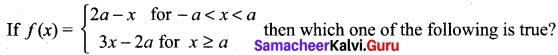(a) f (x) is continuous for all x in R
(b) f(x) is differentiable for all x > a
(c) f(x) is not differentiable at x = a
(d) f (x) is discontinuous at x = a
(c) f(x) is not differentiable at x = a

Question 7.
A number is selected from the set {1, 2, 3, 20}. The probability that the selected number is divisible by 3 or 4 is………..
(a) $$\frac{1}{2}$$
(b) $$\frac{2}{3}$$
(c) $$\frac{2}{5}$$
(d) $$\frac{1}{8}$$
(a) $$\frac{1}{2}$$

Question 8.
Which of the following is not a periodic function with period 2π?
(a) tan x
(b) cos x
(c) sin x
(d) cosec x
(a) tan x

Question 9.
The straight line joining the points (2, 3) and (-1, 4) passes through (α, β) if………..
(a) α + 3β = 11
(b) 3α + β = 11
(c) α + 2β = 7
(d) 3α + β = 9
(a) α + 3β = 11

Question 10.
The minimum and the maximum values of |cos x| -2 are respectively………..
(a) 0 and 2
(b) -2 and 0
(c) -2 and -1
(d) -1 and 1
(c) -2 and -1Question 11.
If A = {(x, y) / y – ex, x ∈ [0, ∞)} and B = {(x, y) / y = sin x, x ∈ [0, ∞)} then n(A ∩ B) is………..
(a) ∞
(b) 1
(c) φ
(d) 0
(d) 0

Question 12.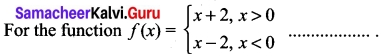(a) $$\underset { x\rightarrow { 2 }^{ – } }{ lim }$$ f(x) = -1
(b) $$\underset { x\rightarrow { 0 } }{ lim }$$ f(x) does not exist
(c) $$\underset { x\rightarrow { 0 }^{ – } }{ lim }$$ f(x) = -1
(d) $$\underset { x\rightarrow { 0 }^{ + } }{ lim }$$ f(x) = 1
(b) $$\underset { x\rightarrow { 0 } }{ lim }$$ f(x) does not exist

Question 13.
If f(x) = x² – 3x, then the points at which f(x) =f'(x) are…………
(a) both irrational
(b) one rational and another irrational
(c) both positive integers
(d) both negative integers
(a) both irrational

Question 14.
The unit vector parallel to the resultant of the vectors $$\hat { i }$$ + $$\hat { j }$$ – $$\hat { k }$$ and $$\hat { i }$$ – 2$$\hat { j }$$ + $$\hat { k }$$ is …………
(a) $$\frac{2 \hat{i}-\hat{j}+\hat{k}}{\sqrt{5}}$$
(b) $$\frac{2 \hat{i}-\hat{j}}{\sqrt{5}}$$
(c) $$\frac{\hat{i}-\hat{j}+\hat{k}}{\sqrt{5}}$$
(d) $$\frac{2 \hat{i}+\hat{j}}{\sqrt{5}}$$
(d) $$\frac{2 \hat{i}+\hat{j}}{\sqrt{5}}$$

Question 15.
It is given that the events A and B are such that P(A) = $$\frac{1}{4}$$, P(A/B) = $$\frac{1}{2}$$ and P(B/A) = $$\frac{2}{3}$$. The P(B) is………..
(a) $$\frac{2}{3}$$
(b) $$\frac{1}{2}$$
(c) $$\frac{1}{6}$$
(d) $$\frac{1}{3}$$
(d) $$\frac{1}{3}$$

Question 16.
If $$\vec { a }, \vec { b }$$ are the position vectors of A and B, then which one of the following points whose position vector lies on AB?
(a) $$\frac{2 \vec{a}+\vec{b}}{2}$$
(b) $$\frac{\vec{a}-\vec{b}}{3}$$
(c) $$\vec { a } + \vec { b }$$
(d) $$\frac{2 \vec{a}-\vec{b}}{2}$$
(a) $$\frac{2 \vec{a}+\vec{b}}{2}$$

Question 17.
If |x + 2| ≤ 8, then x belongs to…………
(a) (6, 10)
(b) (-10, 6)
(c) [6, 10]
(d) [-10, 6]
(d) [-10, 6]Question 18.
The expansion of (1 – x)-2 is ………..
(a) 1 – x + x² – ……
(b) 1 + x + x² + ……
(c) 1 – 2x + 3x² – ….
(d) 1 + 2x + 3x² + ……
(d) 1 + 2x + 3x² + ……

Question 19.
If f : R → R is defined by f(x) = |x| – 5, then the range of f is………..
(a) (-∞, -5)
(b) (-∞, 5)
(c) [-5, ∞)
(d) (-5, ∞)
(c) [-5, ∞)

Question 20.
Which one of the following is not true about the matrix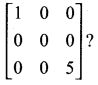(a) an upper triangular matrix
(b) lower triangular matrix
(c) a scalar matrix
(d) a diagonal matrix
(c) a scalar matrix

PART-II

II. Answer any seven questions. Question No. 30 is compulsory. [7 × 2 = 14]

Question 21.
Write the use of horizontal line test.
Horizontal line test is used to check whether a function is one-one, onto or not.

Question 22.
Write the relationship between Permutation and Combination.
Permutation means selection followed by arrangement.
Combination means selection only now selcting Y from ‘n’ things can be done in nCr ways ….(1) (r ≤ n)
Selecting and arranging r from n things can be done in nPr ways ……(2)
‘r’ things can be arranged in r! ways …… (3)
Now (2) = (1) × (3)
(i.e.,) nPr = nCr × r!
nCr = $$\frac{^{n}P_r}{r!}$$Question 23.
Count the number of positive integers greater than 6000 and less than 7000 which are divisible by 5, provided that no digits are repeated.
The required numbers is = 1 × 8 × 7 × 2
= 112

Question 24.
Find the separate equations from a combined equation of a straight line 2x² + xy- 3y² = 0
The combined equation is 2x² +xy – 3y² = 0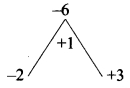Factorizing (2) (-3) = -6
2x² + xy – 3y² = 2x² – 2xy + 3xy – 3y²
= 2x(x – y) + by (x – y) = (2x + by) (x – y)
∴ 2x² + xy – by² = 0 ⇒ (2x + 3y) (x – y) = 0
So the separate equations are 2x + 3y = 0; x – y = 0

Question 25.
Define diagonal and scalar matrices.
A square matrix A = [aij]n × n is called a diagonal matrix
If aij = 0 whenever i ≠ j
A square matrix A = [aij]n × n is called a scalar matrix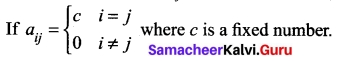Question 26.
Find a unit vector along the direction of the vector $$5 \hat{i}-3 \hat{j}+4 \hat{k}$$
Let $$\hat{a}$$ = $$5 \hat{i}-3 \hat{j}+4 \hat{k}$$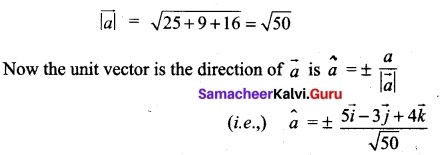Question 27.
Define a continuous function on the closed interval [a, b]
A function f : [a, b] → R is said to be continuous on the closed interval [a, b] if it continuous on the open interval (a, b) and
$$\underset { x\rightarrow { a }^{ + } }{ lim }$$ f(x) = f(a) and $$\underset { x\rightarrow { b }^{ – } }{ lim }$$ f(x) = 1 f(x) = f(b)

Question 28.
Consider the function f(x) = √x, x ≥ 0. Does $$\underset { x\rightarrow { 0 }}{ lim }$$ f(x) exist?
$$\underset { x\rightarrow { 0 }^{ – } }{ lim }$$ √x is does not exist
$$\underset { x\rightarrow { 0 }^{ + } }{ lim }$$ √x = 0
$$\underset { x\rightarrow { 0 } }{ lim }$$ √x is does not exist

Question 29.
An integer is chosen at random from the first ten positive integers. Find the probability that it is multiple of three.
S = {1, 2, 3, 4, 5, 6, 7, 8, 9, 10}
A = {3, 6, 9}
n(S) = 10; n(A) = 3
P(A) = $$\frac{n(A)}{n(S)}$$ = $$\frac{3}{10}$$

Question 30.
Is it correct to say A × A = {(a, a): a ∈ A}? Justify your answer.
It is not correct
Since A × A = {(a, b); a, b ∈ A} is truePART-III

Answer any seven questions. Question no. 40 is compulsory [7 × 3]

Question 31.
A football player can kick a football from ground level with an initial velocity (u) of 80 ft/second. Find the maximum horizontal distance the football travels and at what angle
Take R = $$\frac{u² sin 2α}{g}$$, and g = 32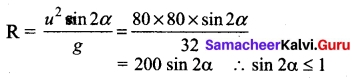Maximum distance = 200ft
The required angle is $$\frac{π}{4}$$

Question 32.
Find the coefficient of x3 in the expansion of (2 – 3x)7.
The general term is (2 – 3x)7 is tr + 1 = 7Cr (2)7 – r (-3x)r
= 7Cr (2)7 – r (-3x)r (x)r
So the co-efficient of xr is 7Cr (2)7 – r (-3)r
To find co-efficient of x³
equate r to 3 (i.e.,) r = 3
∴ co-efficient of x³ is 7Cr (2)7 – 3 (-3)3
= $$\frac{7×6×5}{3×2×1}$$ (2)4 (-27)
= 7 × 5 × (16) (-27)
= -15120

Question 33.
Find the nearest point on the line x – 2y – 5 from the origin.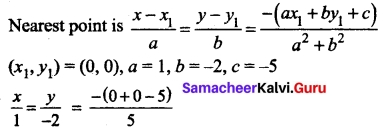x = 1, y = -2
Nearest point is (x1, y1) = (1, -2)

Question 34.
Prove that square matrix can be expressed as the sum of a symmetric matrix and a skew-symmetric matrix.
Let A be a square matrix. Then A = $$\frac{1}{2}$$(A + AT) + $$\frac{1}{2}$$(A – AT)
We know that, (A + AT) is a symmetric, (A – AT) is a skew-symmetric
A can be written as sum of a symmetric skew symmetric matrices.Question 35.
If $$\vec { a }$$ , $$\vec { b }$$, $$\vec { c }$$ are three vectors such that $$\vec { a }$$ + 2$$\vec { b }$$ + $$\vec { c }$$ = 0, and |$$\vec { a }$$|=3, |$$\vec { b }$$| =4, |$$\vec { c }$$| =7, find the angle between $$\vec { a }$$ and $$\vec { b }$$.
$$\vec { a }$$ + 2$$\vec { b }$$ + $$\vec { c }$$ = 0
∴ $$\vec { a }$$ + 2$$\vec { b }$$ = $$\vec { c }$$
squaring on both sides
($$\vec { a }$$ + 2$$\vec { b }$$)² = c²
a² + 4b² + 4$$\vec { a }$$$$\vec { b }$$ = c²
3² + 4(4²) + 4 $$\vec { a }$$ $$\vec { b }$$ = 7²
9 + 64 + 4$$\vec { a }$$ $$\vec { b }$$ = 49
4$$\vec { a }$$ $$\vec { b }$$ = 49 – 73 = -24
$$\vec { a }$$ $$\vec { b }$$ = –$$\frac {24}{4}$$ = -6
|$$\vec { a }$$| |$$\vec { b }$$| cos θ = -6 ⇒ (3) (4) cos θ = -6
⇒ cos θ = –$$\frac {6}{12}$$ = –$$\frac {1}{2}$$ ⇒ θ = π – $$\frac {π}{3}$$ = $$\frac {2π}{3}$$

Question 36.
Examine the continuity of the function cot x + tan x.
Let f (x) = cot x + tan x
cot x is continuous in R – nπ
tan x is continuous in R – (n + 1) $$\frac {π}{2}$$
∴ f(x) is continuous in R – $$\frac {nπ}{2}$$, (n∈Z)

Question 37.
Differentiate y = sin-1 ($$\frac {1-x²}{1+x²}$$)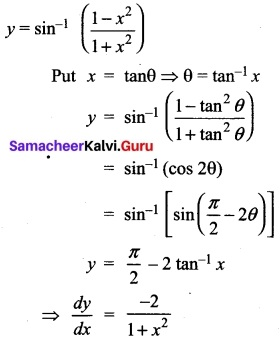Question 38.
find $$\frac {dy}{dx}$$ if x = a (t – sin t), y = a(1 – cos t)
x = a (t – sin t) ⇒ $$\frac {dy}{dx}$$ = a(1 – cos t)
y = a(1 – cos t) ⇒ $$\frac {dy}{dt}$$ = a(sin t)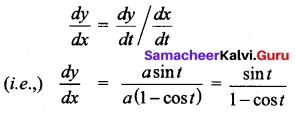Question 39.
Evaluate $$\int { (x+3) } \sqrt { x+2 }$$ dx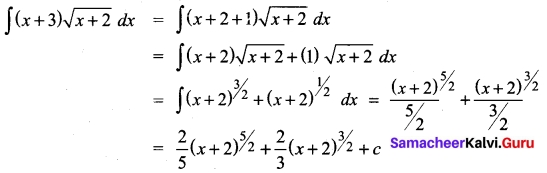Question 40.
Construct a suitable domain X such that f : X → N defined by/(n) = n + 3 to be one to one and onto.
f : X → N is defined by f(x) = n + 3
Since f is one-one and onto function
suitable Domain is (-2, 1,0} ∪ N (or) {-2, -1, 0, 1, 2}

PART- IV

IV. Answer all the questions. [7 x 5 = 35]

Question 41 (a).
For the given base curve y = sin x, draw y = $$\frac {1}{2}$$ sin 2x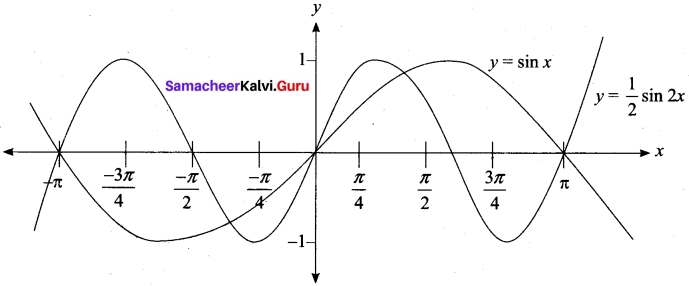[OR]

(b) Write any five different forms of an equation of a straight line.
(i) Slope intercept form : y = mx + c, c ≠ 0
(ii) Point and slope form : y – y1 = m (x – x1)
(iii) Two Point form : $$\frac {y – y_1}{y_{2}-y_1}$$ $$\frac {x – x_1}{x_{2}-x_1}$$
(iv) Intercept form : $$\frac {x}{a}$$ + $$\frac {y}{b}$$ = 1
(v) Normal form : x cos α + y sin α

Question 42 (a).
Solve the equation $$\sqrt {6-4x-x²}$$ = x + 4
Squaring both sides
6 – 4x – x² = (x + 4)²
(i.e.,) x² + 6x + 5 = 0 ⇒ (x + 1) (x + 5) = 0
⇒ x = -1, x = -5
But x ≥ – 4
⇒ x = -1

[OR]

(b) Prove that in any ΔABC, Δ = $$\sqrt{s(s-a)(s-b)(s-c)}$$, where s is the semi-perimeter of ΔABC.
Δ = $$\frac {1}{2}$$ ab sin C = $$\frac {1}{2}$$ ab (2sin $$\frac {C}{2}$$ cos $$\frac {C}{2}$$)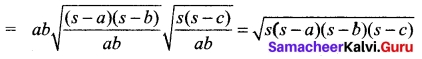Question 43 (a).
State and prove any one of the Napier’s formulae.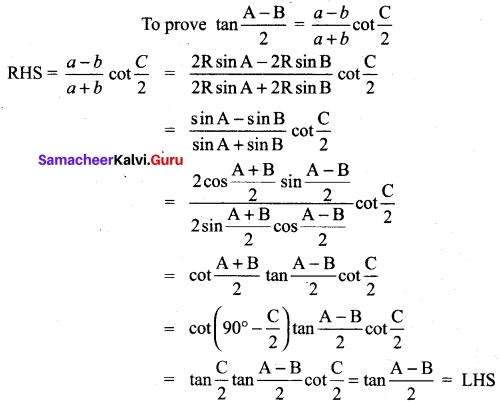[OR]

(b) Do the limit of the function $$\frac {sin(x-\left\lfloor x \right\rfloor)}{x – \left\lfloor x \right\rfloor}$$ exist as x → 0? State the reasons for your answer.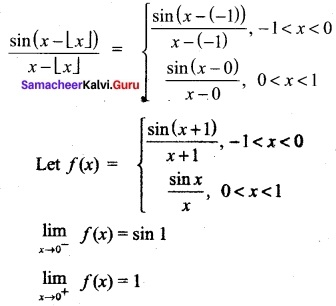So, the limit does not exist.

Question 44. (a)
Prove that for any natural number n, an – bn is divisible by a – b, where a > b.
a = a – b + b
So, an =[a- b + b]n = [(a -b) + b]n
= nC0 (a – b)n + nC1 (a – b)n-1b1 + nC2 (a – b)n-2b² + ……. + nCn-1, (a – b) bn-1+ nCn(bn)
⇒ an – bn = (a – b)n + nC1 (a – b)n-1b + nC2 (a – b)n-2b²) + …….. + nCn-1 (a – b) bn-1
= (a – b) [(a – b)n-1 + nC1 (a – b)n-2b + nC2 (a – b)n-3b² + …….. + nCn-1 bn-1]
= (a – b) [an integer]
⇒ an – bn If is divisible by(a – b).

[OR]

(b) Evaluate: $$\int \frac{2 x+4}{x^{2}+4 x+6}$$
Let 2x + 4 = A $$\frac {d}{dx}$$ (x² + 4x + 6) + B
(i.e.,) 2x + 4 = A(2x + 4) + B
Equating the coefficient of x and constant term we get
A = 1, B = 0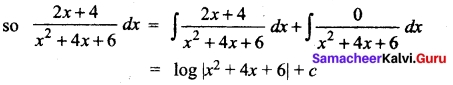Question 45 (a).
Prove that $$\sqrt{x^{3}+7}-\sqrt{x^{3}+4}$$ is approximately equal to $$\frac {1}{x²}$$when x is large.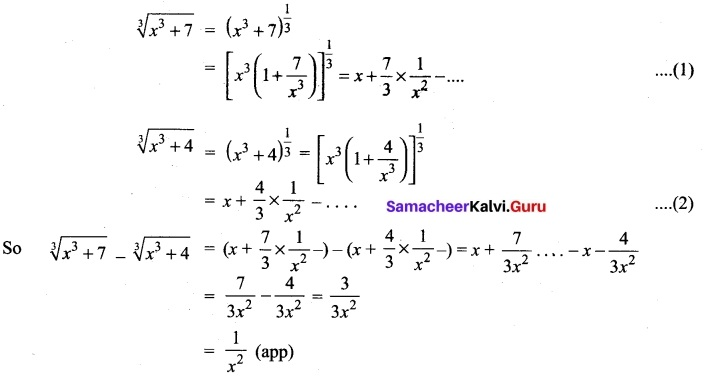[OR]

(b) Find the unit vectors perpendicular to each of the vectors $$\vec { a }$$ + $$\vec { b }$$ and $$\vec { a }$$ – $$\vec { b }$$ where $$\vec { a }$$ = $$\hat { i }$$ + $$\hat { j }$$ + $$\hat { j }$$ and $$\vec { b }$$ = $$\hat { i }$$ + 2$$\hat { j }$$ + 3$$\hat { k }$$.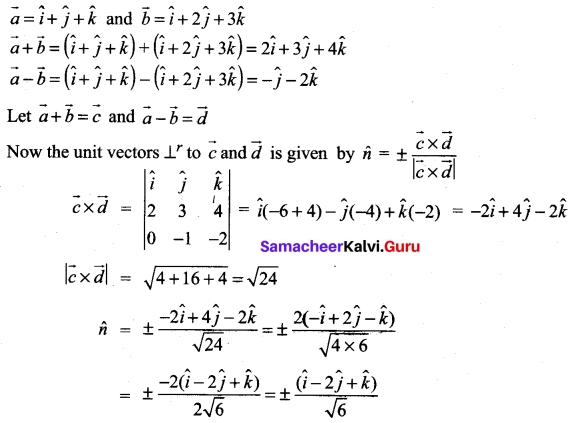Question 46 (a).
Find $$\frac {d²y}{dx}$$ if x² + y² = 4
we have x² + y² = 4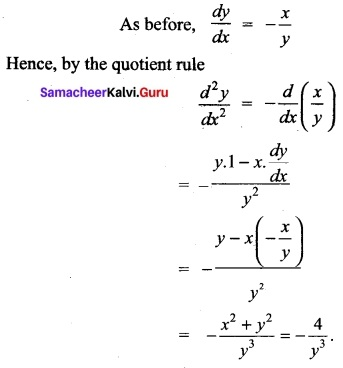[OR]

(b) The chances of X, Y and Z becoming managers of a certain company are 4 : 2 : 3. The probabilities that bonus scheme will be introduced if X, Y and Z become managers are 0.3, 0.5 and 0.4 respectively. If the bonus scheme has been introduced, what is the probability that Z was appointed as the manager?
Given X : Y : Z = 4 : 2 : 3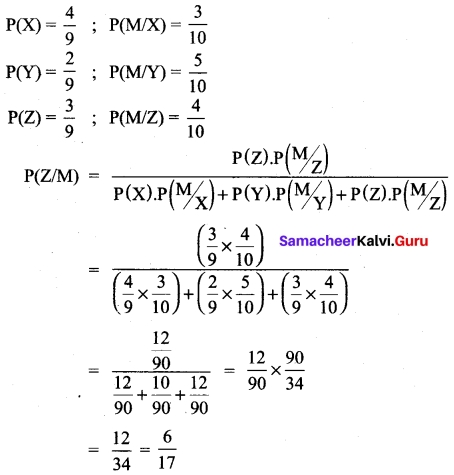Question 47 (a).
Prove that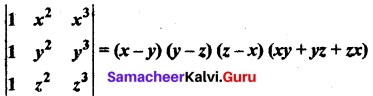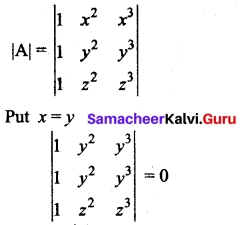∴ (x – y) is a factor
Similarly (y – z)(z – x) are also factors.
The other factor is k(x² + y² + z²) + l(xy + yz + zx)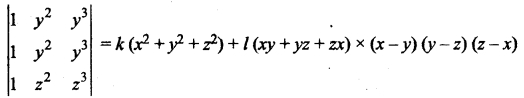Putting x = 0, y = 1, z = 2 ⇒ 5k + 2l = 2 …. (1)
Putting x = 0, y = – 1, z = 1 ⇒ 2k – l = -1 …. (2)
from (1) & (2) k = 0, l = -1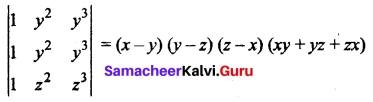[OR]

(b) Evaluate $$\int \sqrt{x^{2}+x+1}$$ dx